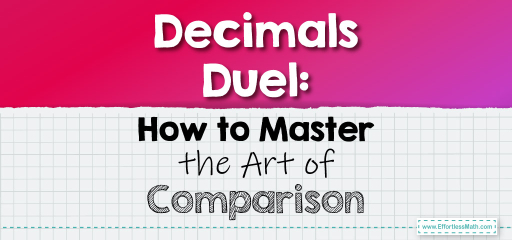# Decimals Duel: How to Master the Art of Comparison

Decimals represent parts of a whole, and comparing them is essential in various mathematical and real-world scenarios. When comparing decimals, it's crucial to look at each place value, starting from the leftmost digit, and work our way to the right. Let's delve into the techniques to compare decimals effectively.## Comparing Decimals

### Example 1:

Decimals to Compare: $$0.6$$ and $$0.58$$

Comparison:

Starting from the tenths place, the digit in $$0.6$$ is $$6$$, while in $$0.58$$, it’s $$5$$. Since $$6$$ is greater than $$5$$, $$0.6$$ is greater than $$0.58$$.

$$0.6 > 0.58$$

The Absolute Best Book for 5th Grade Students

### Example 2:

Decimals to Compare: $$0.72$$ and $$0.725$$

Comparison:

Both numbers have $$7$$ in the tenths place. Moving to the hundredths place, both have $$2$$. However, $$0.725$$ has an additional digit in the thousandths place, which makes it larger.

$$0.725 > 0.72$$

When comparing decimals, it’s essential to consider each place value. If the decimals have different numbers of digits, remember that trailing zeros (like in $$0.450$$) don’t affect the value. With practice, you’ll become adept at comparing decimals swiftly and accurately!

### Practice Questions:

1. Which is greater: $$0.45$$ or $$0.450$$?

2. Compare $$0.9$$ and $$0.89$$. Which is larger?

3. Determine the smaller decimal between $$0.123$$ and $$0.13$$.

4. Which is greater: $$0.505$$ or $$0.51$$?

5. Compare $$0.67$$ and $$0.670$$. Which is smaller?

A Perfect Book for Grade 5 Math Word Problems!

1. They are equal.

2. $$0.9$$

3. $$0.123$$

4. $$0.51$$

5. They are equal.

The Best Math Books for Elementary Students

### What people say about "Decimals Duel: How to Master the Art of Comparison - Effortless Math: We Help Students Learn to LOVE Mathematics"?

No one replied yet.

X
51% OFF

Limited time only!

Save Over 51%

SAVE $15 It was$29.99 now it is \$14.99